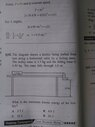# Finding the Kinetic energy of a Trolley being pulled by a falling weight via a cable

haha0p1
Homework Statement:
The diagram shows a trolley being pulled from rest along a horizontal table by a falling mass. The trolley mass is 1.5 kg and the falling mass is 0.50 kg. The mass falls through 1.0m. what is the maximum kinetic energy of the trolley?
Relevant Equations:
Ek= 1/2 mv²
Ek of the trolley = 1/2×1.5×v²
How are we going to find the v (velocity) to put into the formula?

#### Attachments

•IMG_20230102_120913.jpg
35.6 KB · Views: 21

Homework Helper
Gold Member
What form of energy does the falling mass have at the beginning of the experiment? What form of energy does the falling mass have at the end?

Remember that Energy is conserved, but it can change form.

•haha0p1
haha0p1
Before the fall, the falling mass has gravitational potential energy and when the mass is falling, that gravitational potential energy is changing to Kinetic energy So,
Gravitational potential energy=mg∆h=0.5×10×1=5J
Kinetic energy=Gravitational potential energy
5=1/2 mv²=1/2 ×0.5×v²= 0.25×v²
v=√5÷0.25=4.5
Using the value of v=4.5 for the kinectic energy formula of the trolley, we have:
Ek=1/2×1.5×4.5²=15 J. My answer is still coming 15J while the correct answer is 3.7J. Kindly tell where am I going wrong?

Last edited:
Gold Member
Before the fall, the falling mass has gravitational potential energy and when the mass is falling, that gravitational potential energy is changing to Kinetic energy So,
Gravitational potential energy=mg∆h=0.5×10×1=5J
Kinetic energy=Gravitational potential energy
5=1/2 mv²=1/2 ×0.5×v²= 0.25×v²
v=√5÷0.25=4.5
Using the value of v=4.5 for the kinectic energy formula of the trolley, we have:
Ek=1/2×1.5×4.5²=15 J. My answer is still coming 15J while the correct answer is 3.7J. Kindly tell where am I going wrong?

5=1/2 mv²=1/2 ×0.5×v²= 0.25×v²

I'm pretty sure the 0.5 I've highlighted in red is where you went wrong.
The change in potential energy to kinetic energy imparted by the falling weight is transferred to both the trolley and the weight.

•nasu and haha0p1
haha0p1
5=1/2 mv²=1/2 ×0.5×v²= 0.25×v²

I'm pretty sure the 0.5 I've highlighted in red is where you went wrong.
The change in potential energy to kinetic energy imparted by the falling weight is transferred to both the trolley and the weight.
5=1/2 mv²=1/2 ×(0.5+1.5)×v²= 1×v²
v=√5=2.24
Using the value of v=2.24 for the kinectic energy formula of the trolley, we have:
Ek=1/2×1.5×2.24²=3.7J
Yes, Now I am getting the right answer.

•berkeman and OmCheeto